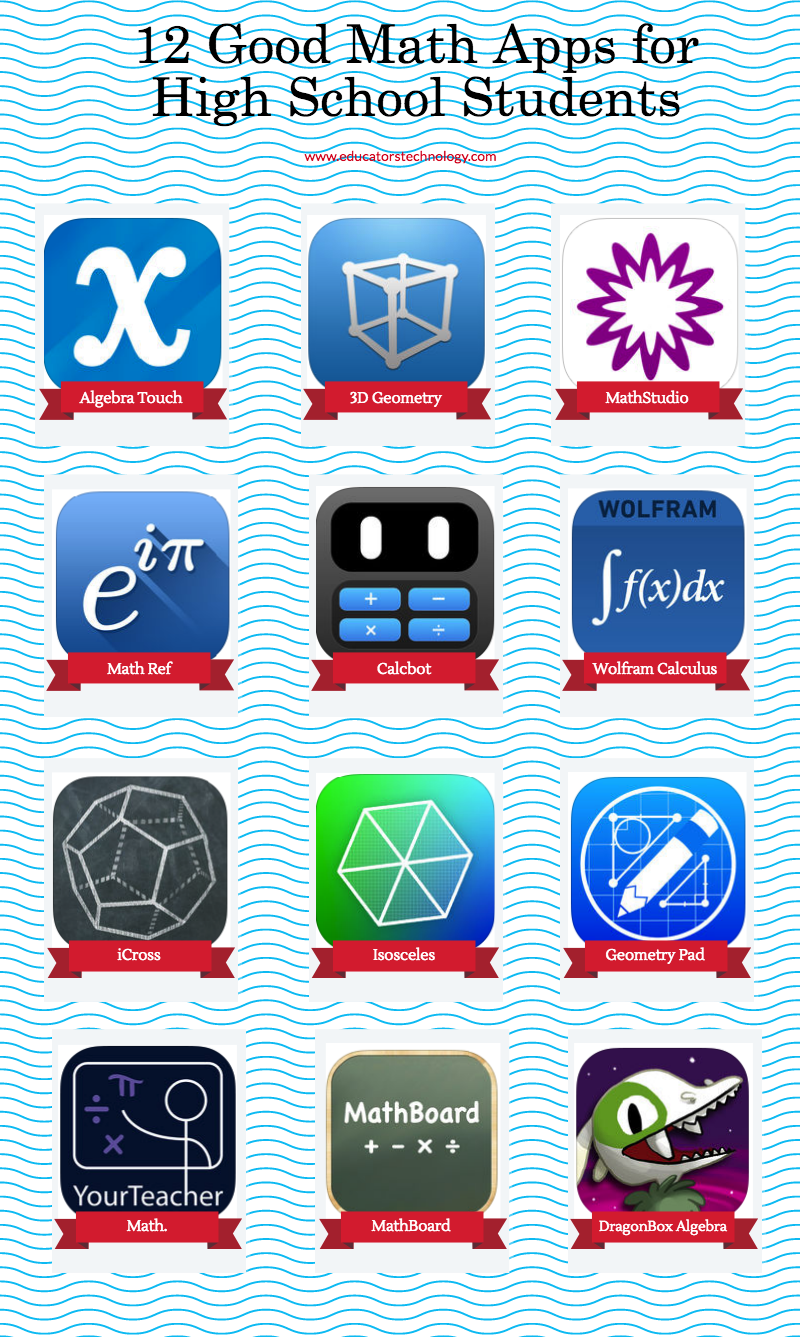# 12 Good Math Apps for High School Students

This is our first post in a series of posts which will be dedicated entirely to educational iPad apps to use with high school students. Today's list, we curated from iTunes app store, is about math apps. We included apps that cover five main areas: algebra, geometry, calculus, graphing and calculation. We invite you to check them out and share with us your feedback. Links to the apps are under the visual.1- Algebra Touch
‘Have you forgotten most of your algebra? Algebra Touch will refresh your skills using touch-based techniques built from the ground up’

2- 3D Geometry
‘3D Geometry is a app designed for observing and manipulating geometrical figures in three dimensional space.’

3- MathStudio
‘Download the free online version of MathStudio, a full featured scientific calculator with hundreds of mathematical functions that cover Basic Math, Pre-Algebra, Algebra, Trigonometry, Precalculus, Calculus, Statistics, Finite Math, Linear Algebra and Statistics.’

4- Math Ref
‘Math Ref is an award winning education app. Browse over 1,400 formulas, figures, and examples to help you with math, physics, chemistry and more. Tools such as a unit converter, quadratic solver, and triangle solver help you perform common calculations fast.’

5- Calcbot
‘Calcbot is an intelligent calculator and unit converter for the rest of us. Featuring an easy-to-read history tape, expression view, intuitive conversion, and much more!’

6- Wolfram Calculus Course Assistant
‘Taking calculus? Then you need the Wolfram Calculus Course Assistant. This definitive app for calculus—from the world leader in math software—will help you work through your homework problems, ace your tests, and learn calculus concepts.’

7- iCross
‘iCrosss is a unique educational application which will help you learn solid geometry in an easy and funny way.’

8- Isosceles
‘Isosceles is the perfect geometry drawing tool for students, teachers, and professionals.’